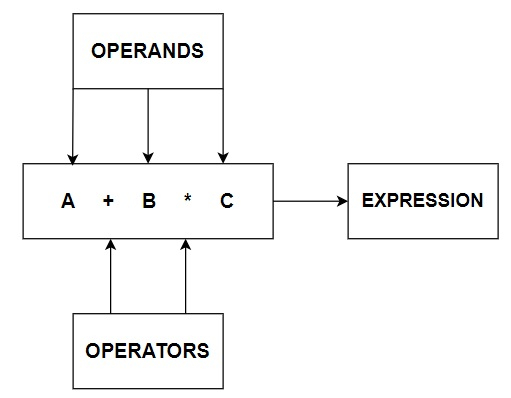# What are the assignment operators in Java?

## OPERATORS

In computer programming we often need to perform some arithmetical or logical operations. In such circumstances, we need operators to perform these tasks. Thus, an Operator is basically a symbol or token, which performs arithmetical or logical operations and gives us meaningful result. The values involved in the operation are called Operands.

Here is a basic Pictorial representation of Operators.## ASSIGNMENT OPERATORS

Assignment Operators are a part of Binary Operators which are included in Arithmetical Operators. They are used to assign values to a variable. The left hand operand of the assignment operator indicates variable and the right hand operand indicates value. The data type of the value on the right must be the same as that of the variable, otherwise will result in Compilation error. For example: =, + =, - =, / =, >> =, ^ =, ! = are few examples of Assignment Operators.

Below are the types of Assignment operators available to use in Java.

• = is a simple assignment operator which assigns values from right operands to left operand.

For Example, A = 2 will assign value 2 into A

• += is an Add assignment operator which adds right operand to the left operand and assigns the result to the left operand.

For Example, A += B is equivalent to A = A + B

• -= is a Subtract assignment operator which subtracts right operand from the left operand and assigns the result to the left operand.

For Example, A -= B is equivalent to A = A – B

• *= is a Multiply assignment operator which multiplies right operand with the left operand and assigns the result to the left operand.

For Example, A *= B is equivalent to A = A * B

• /= is a Divide assignment operator which divides left operand with the right operand and assigns the result to the left operand.

For Example, A /= B is equivalent to A = A / B

• %= is a Modulus assignment operator which takes modulus using left and right operands and assigns the result to the left operand.

For Example, A %= B is equivalent to A = A % B

• <<= is a Left shift assignment operator which left shifts the left operand by number of positions given by the right operand.

For Example, A <<= 2 is same as A = A << 2

• >>= is a Right shift assignment operator which right shifts the left operand by number of positions given by the right operand.

For Example, A >>= 2 is same as A = A >> 2

• &= is a Bitwise AND assignment operator which adds Bitwise right operand to Bitwise Left operand and assigns the result to the left operand.

For Example, A &= B is same as A = A & B

• ^= is a Bitwise XOR assignment operator which returns bit by bit XOR values of right operand and assigns the result to the left operand.

For Example, A ^= 2 is same as A = A ^ 2

• |= is a Bitwise OR assignment operator which returns bit by bit OR values of right operand and assigns the result to the left operand.

For Example, A |= 2 is same as A = A | 2

### Example

Let’s see how to implement all above Assignment Operators in a Java Code.

public class AssignmentOperators {// public class declaration
public static void main(String[] args){ // main function declaration
int a,b = 2; // variable declaration and initialization
a = 11;
System.out.println(" = Operator : a = " + a); // simple assignment operator
a += b;
System.out.println(" += Operator : a += b is : " + a); // add assignment operator
a -= b;
System.out.println(" -= Operator : a -= b is : " + a); // subtract assignment operator
a *= b;
System.out.println(" *= Operator : a *= b is : " + a); // multiply assignment operator
a /= b;
System.out.println(" /= Operator : a /= b is : " + a); // division assignment operator
a %= b;
System.out.println(" %= Operator : a %=b is : " + a); // modulus assignment operator
a <<= 2;
System.out.println(" <<= Operator : a <<= 2 is : " + a); // Left shift assignment operator
a >>= 2;
System.out.println(" >>= Operator : a >>= 2 is : " + a); // Right shift assignment operator
a &= b;
System.out.println(" &= Operator : a &= b is : " + a); // Bitwise AND assignment operator
a |= 2;
System.out.println(" |= Operator : a |= 2 is : " + a); // Bitwise OR assignment operator
a ^= 2;
System.out.println(" ^= Operator : a ^= 2 is : " + a); // Bitwise XOR assignment operator
}
}


### Output

= Operator : a = 11
+= Operator : a += b is : 13
-= Operator : a -= b is : 11
*= Operator : a *= b is : 22
/= Operator : a /= b is : 11
%= Operator : a %=b is : 1
<<= Operator : a <<= 2 is : 4
>>= Operator : a >>= 2 is : 1
&= Operator : a &= b is : 0
|= Operator : a |= 2 is : 2
^= Operator : a ^= 2 is : 0


I hope you have understood all concepts behind Assignment Operators in Java. Thanks for reading the article.